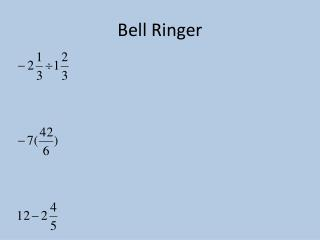DownloadDownload PresentationBell Ringer

# Bell Ringer

Télécharger la présentation## Bell Ringer

- - - - - - - - - - - - - - - - - - - - - - - - - - - E N D - - - - - - - - - - - - - - - - - - - - - - - - - - -
##### Presentation Transcript

1. Bell Ringer

2. Solving 1-Step Integer Equations Objective: To solve one-step integer equations using addition, subtraction, multiplication, and division

3. Solve an Equation • To find all values of a variable that make an equation true

4. A one-step equation is as straightforward as it sounds. You will only need to perform one step in order to solve the equation.

5. Solving One-Step Equations • Inverse Operation • Operations that “undo” each other • For example, addition “undoes” subtraction and subtraction “undoes” addition. • Multiplication is the inverse of division

6. Solving Equations Using Addition or Subtraction: • If a number has been addedto the variable, subtract that number from both sides of the equation. • If a number has been subtracted from the variable, add that number to both sides of the equation.

7. Example 1: Solve m + 7 = 11 m + 7 = 11 To undo the addition of 7, subtract 7 from both sides of the equal sign. - 7 -7 m = 4 Check the solution. m + 7 = 11 Replace the variable with your answer. (4) + 7 = 11  11= 11

8. Example 2:Solve -6 = m - 4 -6 = m - 4 To undo the subtraction of 4, add 4 to both sides of the equal sign. +4 +4 -2 = m Check the solution. -6 = m - 4 Replace the variable with your answer. -6 = (-2) - 4  -6 = -6

9. Practice j - 3 = -12 -4 + r = 26 +4 +4 +3 +3 r = 30 j = - 9

10. Solving Equations Using Multiplication or Division • If a variable has been multipliedby a nonzero number, divide both sides by that number. • If a variable has been divided by a number, multiply both sides by that number.

11. Example 3: Solve 3y = 261 3y = 261 To undo the multiplication by 3, divide 3 from both sides of the equal sign. 3 3 y = 87 Check the solution. 3y = 261 Replace the variable with your answer. 3(87) = 261  261= 261

12. Example 4: Use the multiplication property of - 1 -w = 42 -1w = 42 To undo the multiplication of -1 , divide both sides by -1. -1 is being multiplied to w -1 -1 w = -42 Check the solution. -w = 42 Replace the variable with your answer. -(-42) = 42  42= 42

13. Practice -6x = -36 b = 12 (-4) (-4) -6 -6 -4 x = 6 b = -48

14. Practice: Solve and Check • 1. x-(-8)=4 2. -5x=19 • 3. x/7=-12

15. Practice: Solve and Check • 4. x+7=-2 5. -4x=6

16. Practice: Solve and Check • 6. 9=5+x 7. -5.1+x=-7.25